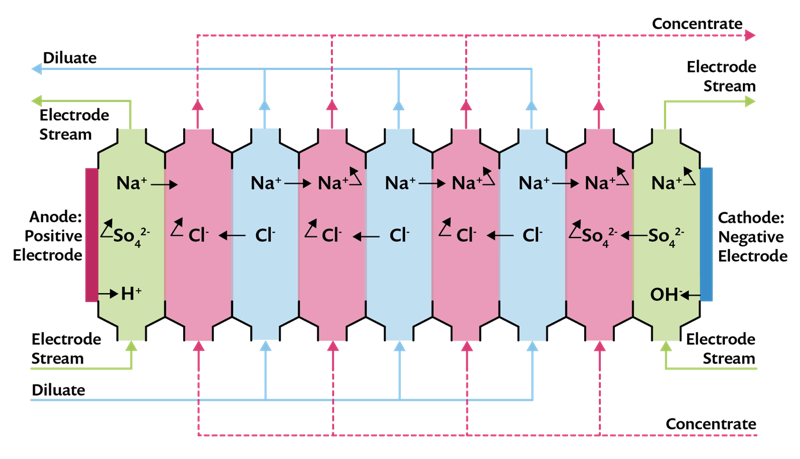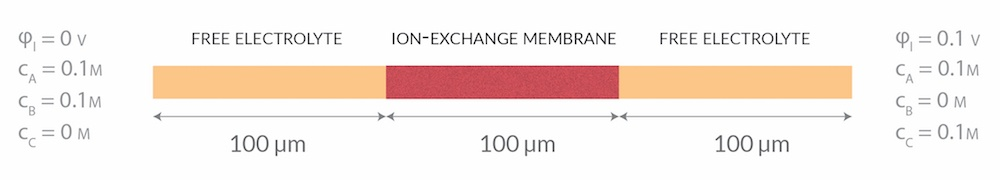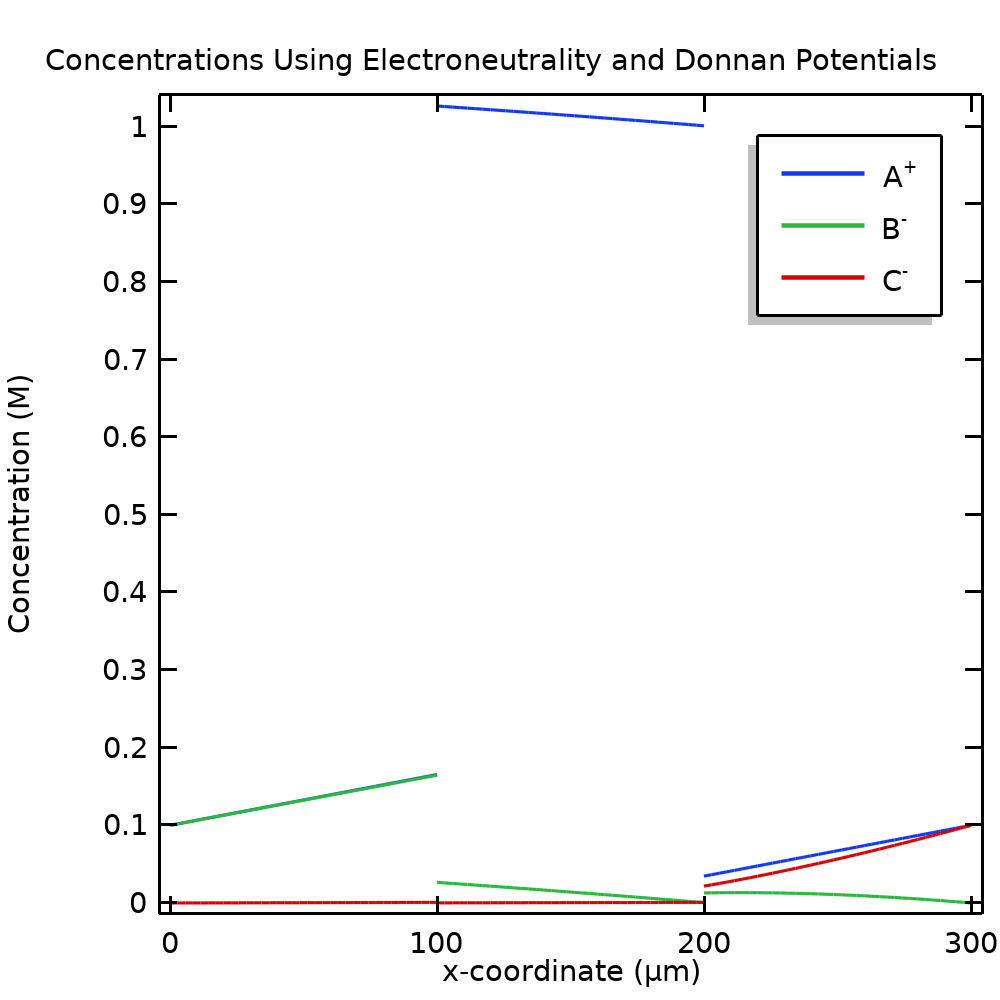# 如何模拟离子交换膜和唐南电位

2018年 8月 29日

### Nernst-Planck-Poisson 方程

{SO}
_3^-

(1)

\nabla \cdot (-\epsilon \nabla \phi_l) = \rho

(2)

\rho = F \sum_i^N z_i c_i + \rho_\textrm
{fix}

(3)

\mu_i = RT \mathrm{log}(\frac{c_i}{c_{i,\textrm{ref}}}) + Fz_i\phi_l

(4)

\mathbf{J}_i = – \mathrm{mob}_i c_i \nabla \mu_i

(5)

\textrm{mob}_i = \frac{D_i}{RT}

(6)

\nabla \cdot \mathbf{J}_i =0

### 用 NPP 方程模拟离子交换膜B和 C浓度的边界条件决定了这些物质的整体通量方向，但电场的方向解释了为什么 B以较快的速率向右侧传输，而 C以较慢的速率向左侧传输。当增加膜电荷时，我们可以看到 B的通量减少（仔细观察可发现 C也是如此）；也就是说，增加膜电荷会导致 B和 C在膜传递中受到的阻塞力变大。还应该注意的是，阻塞只是部分原因。### 唐南电位条件和电中性简介\phi_l,u-\phi_l,d = -\frac{RT}{z_i}\textrm{log}\frac{c_i,u}{c_i,d}

\nabla \cdot \sum_i^N z_iF\mathbf{J}_i =0

（为方便起见，我们还将总和与 F 相乘，得到一个单位为 A/m2的表达式；即总电流密度。这样，纽曼边界条件可以用这个单位来表示。）### 进一步提高模型收敛性

\nabla \cdot (z_A F\mathbf{J}_A) = \nabla \cdot (z_A^2 F^2 \textrm{mob}_A c_A \nabla \phi_l) = \nabla \cdot (\sigma_l \nabla \phi_l) = 0

c_A = -\frac{z_\textrm{fix}c_\textrm{fix}}{z_A}

\sigma_l = z_A^2 F^2 \textrm{mob}_A c_A

### 后续操作

Nafion 是 The Chemours Company FC, LLC. 的注册商标。